# AP Calculus BC Practice Test 38

### Test Information10 questions20 minutes

Calculator Disallowed

1. Given the equation y = 3 sin2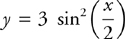, what is an equation of the tangent line to the graph at x = π?

2. Which of the following statements about the series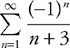is true?

3.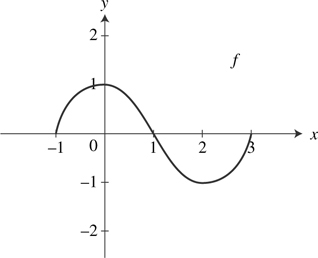The graph of f for -1 ≤ x ≤ 3 consists of two semicircles, as shown above. What is the value of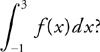4. The series expansion for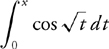is

5. If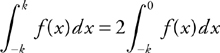for all positive values of k, then which of the following could be the graph of f ?

6. Which of the following is the Taylor series for f (x) = x2 cos x about x = 0?

7.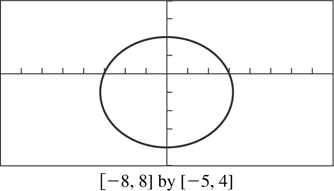The graph of the polar curve r = 3 - sin θ is shown above. Which of the following expressions gives the area of the region enclosed by the curve?

8. What are all values of x for which the series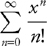converges?

9.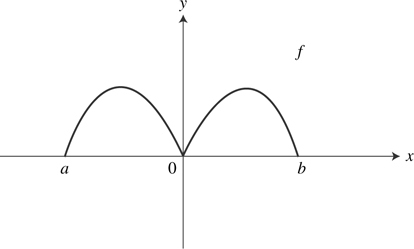The graph of f is shown above, and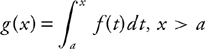. Which of the following is a possible graph of g ?

10. The series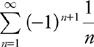satisfies the hypotheses of the alternating series test, i.e.,, and If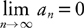S is the sum of the series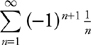and sn is the nth partial sum, what is the minimum value of n for which the alternating series error bound guarantees that |S - sn | < 0.1?